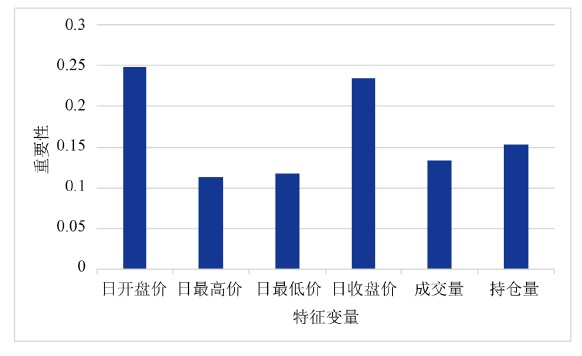基于Xgboost算法的国际期货涨跌预测分析Analysis of the Rise and Fall of International Futures Based on Xgboost Algorithm

• 全文下载: PDF(601KB)    PP.211-220   DOI: 10.12677/FIN.2018.85025
• 下载量: 312  浏览量: 1,594   科研立项经费支持

Based on an efficient and complex xgboost algorithm, a classification prediction model was con-structed to train and test the daily transaction data of international futures in the past three years. The model traverses all parameter combinations through the reference tool to obtain the optimal parameters. Then, it is compared with decision tree, random forest, support vector machine algo-rithm, and combined with multiple evaluation indicators for comprehensive evaluation. Experi-ments show that the indicators of the model constructed by the xgboost algorithm are higher than other algorithms, and the overall prediction ability is better. At the same time, it also provides an effective new method for forecasting futures prices.

1. 引言

2. 相关研究文献评述

Xgboost算法是2016年由陈天奇  博士提出的，该算法已经在众多领域取得了优异的成绩。张昊  将xgboost算法应用于商品推荐中，基于阿里巴巴的真实用户数据进行建模，得到了较准确的预测结果，对个性化推荐系统的完善具有重要的意义。蒋晋文和刘伟光  将xgboost算法应用与制造业质量预测中，处理了制造业生产过程中积累的大量数据，实现了准确预测产品质量的目的。李想  基于xgboost算法成功设计出了超越沪深300指数的超额收益率的多因子量化选股方案，并与随机森林、支持向量机进行了对比，验证了xgboost算法的稳定性与效果。

3. 数据分析

(一) 数据描述

(二) 缺失值填充Table 1. Introduction to the characteristics of the data set

(三) 特征工程

1) 特征筛选

2) 相关性分析

$r=\frac{\underset{i=1}{\overset{n}{\sum }}\left({X}_{i}-\stackrel{¯}{X}\right)\left({Y}_{i}-\stackrel{¯}{Y}\right)}{\sqrt{\underset{i=1}{\overset{n}{\sum }}{\left({X}_{i}-\stackrel{¯}{X}\right)}^{2}}\sqrt{\underset{i=1}{\overset{n}{\sum }}{\left({Y}_{i}-\stackrel{¯}{Y}\right)}^{2}}}$ (1)Table 2. Correlation coefficient between attributes

(三) 归一化处理

${X}_{norm}=\frac{X-{X}_{\mathrm{min}}}{{X}_{\mathrm{max}}-{X}_{\mathrm{min}}}+\sigma$ (2)

4. 分类建模

(一) Xgboost算法

Xgboost算法实现步骤如下：

1) Boosting模型构建

$\varnothing \left({X}_{i}\right)=\underset{k=1}{\overset{K}{\sum }}{f}_{k}\left({X}_{i}\right),{f}_{k}\in F$ (3)

2) 构建目标函数

$\begin{array}{l}L\left(\varnothing \right)=\underset{i}{\sum }l\left({\stackrel{^}{y}}_{i},{y}_{i}\right)+\underset{k}{\sum }\Omega \left({f}_{k}\right)\\ \text{where}\Omega \left(f\right)=\gamma T+\frac{1}{2}\lambda {‖\omega ‖}^{2}\end{array}$ (4)

3) 训练目标函数

${L}^{\left(t\right)}=\underset{i=1}{\overset{n}{\sum }}l\left({y}_{i},{\stackrel{^}{y}}^{\left(t-1\right)}+{f}_{t}\left({X}_{i}\right)\right)+\Omega \left({f}_{t}\right)$ (5)

4) 目标函数二阶泰勒展开

$\begin{array}{l}{L}^{\left(t\right)}\simeq \underset{i=1}{\overset{n}{\sum }}\left[l\left({y}_{i},{\stackrel{^}{y}}^{\left(t-1\right)}\right)+{g}_{i}{f}_{t}\left({X}_{i}\right)+\frac{1}{2}{h}_{i}{f}_{t}^{2}\left({X}_{i}\right)\right]+\Omega \left({f}_{t}\right)\\ \text{where}{g}_{i}={\partial }_{{\stackrel{^}{y}}^{\left(t-1\right)}}l\left({y}_{i},{\stackrel{^}{y}}^{\left(t-1\right)}\right)\text{and}{h}_{i}={\partial }_{{\stackrel{^}{y}}^{\left(t-1\right)}}^{2}l\left({y}_{i},{\stackrel{^}{y}}^{\left(t-1\right)}\right)\end{array}$ (6)

5) 去掉常数项(7)

6) 正则项展开

${\stackrel{˜}{L}}^{\left(t\right)}\simeq \underset{i=1}{\overset{n}{\sum }}\left[{g}_{i}{f}_{t}\left({X}_{i}\right)+\frac{1}{2}{h}_{i}{f}_{t}^{2}\left({X}_{i}\right)\right]+\gamma T+\frac{1}{2}\lambda \underset{j=1}{\overset{T}{\sum }}{\omega }_{j}^{2}$ (8)

${\stackrel{˜}{L}}^{\left(t\right)}\text{=}\underset{j=1}{\overset{T}{\sum }}\left[\left(\underset{i\in {I}_{j}}{\sum }{g}_{i}\right){\omega }_{j}+\frac{1}{2}\left(\underset{i\in {I}_{j}}{\sum }{h}_{i}+\lambda \right){\omega }_{j}^{2}\right]+\gamma T$ (9)

${\omega }_{j}^{*}=-\frac{{\sum }_{i\in {I}_{j}}{g}_{i}}{{\sum }_{i\in {I}_{j}}{h}_{i}+\lambda }$ (10)

7) 目标函数最优解

${\stackrel{˜}{L}}^{\left(t\right)}\left(q\right)=-\frac{1}{2}\underset{j=1}{\overset{T}{\sum }}\frac{{\left({\sum }_{i\in {I}_{j}}{g}_{i}\right)}^{2}}{{\sum }_{i\in {I}_{j}}{h}_{i}+\lambda }+\lambda T$ (11)

(二) 其他分类算法

(三) 评价指标

$\text{AUC}=\frac{1}{2}\underset{i=1}{\overset{m-1}{\sum }}\left({x}_{i+1}-{x}_{i}\right)\ast \left({y}_{i}+{y}_{i+1}\right)$ (12)

$\text{ACC}=\frac{\text{TP}+\text{TN}}{\text{TP}+\text{FN}+\text{FP}+\text{TN}}$ (13)

$\text{Recall}=\frac{\text{TP}}{\text{TP}+\text{FN}}$ (14)

$\text{Precesion}=\frac{\text{TP}}{\text{TP}+\text{FP}}$ (15)

$\text{F1}=\frac{2×\text{Recall}×\text{Precesion}}{\text{Recall}+\text{Precesion}}$ (16)

$\left({x}_{i},{y}_{i}\right)$ 表示ROC曲线上某个点的坐标，TP表示被判定为正样本且事实也是正样本的数量，TN表示被判定为负样本且事实也是负样本的数量，FP表示被判定为正样本但事实是负样本的数量，FN表示被判定为负样本但事实是正样本的数量。同时，对于数据量较大的数据集，运行时间也是评价指标。本实验均在个人PC上完成，用python语言的time函数记录运行时间。由于每次代码运行时间有差别，所以本文取10次运行时间的均值。

5. 实验

(一) 模型调参优化

Xgboost算法的参数分为三类，分别是一般参数、提升参数、学习参数。提高模型的关键是优化该算法的提升参数。为了避免人为尝试调参带来的疏漏，本文采用sklearn.model_selection库中内置函数GridSearchCV来进行辅助调参，GridSearchCV用于遍历所有参数组合，通过交叉验证确定最佳效果参数，这里采用十折交叉验证。具体的调参步骤如下：

1) 通过GridSearchCV遍历设定的学习率取值范围，得出最佳“学习率”，然后根据十折交叉验证来确定“最佳树的个数”。

2) 确定好“学习率”和“最佳树个数”后，调节树的相关参数。首先，给出max_depth和min_child_weight参数组合范围，遍历其所有取值，得出其最佳参数组合。然后，给出colsample_bytree和subsample参数组合范围，遍历其所有值，得出其最佳参数。最后固定以上参数，再得出“最佳树个数”。

3) 利用以上调节好的参数，为了进一步避免过拟合，调节正则化参数，这里我们调节L1正则化参数“alpha”，同样，设定范围，遍历其所有取值，得出最佳参数。然后，再得出“最佳树个数”。

4) 利用步骤3得出的“最佳树个数”，以及之前调好的所有参数，再将学习率变小。得出最佳参数组合。

(二) 模型结果对比Table 4. Comparison of modeling resultsFigure 1. Characteristic variable importance score

(三) 特征重要性分析

6. 结语

  李绍. 运用概率论和数理统计理论研究股市的内在规律[J]. 统计与决策, 1999(2): 16-17.  魏宇. 沪深300股指期货的波动率预测模型研究[J]. 管理科学学报, 2010, 13(2): 66-76.  Wang, C.Y. (2016) Forecast on Price of Agricultural Futures in China Based on ARIMA Model. Asian Agricultural Research, 8, 9-12, 16.  董贺. 基于国内商品期货的量化投资优化策略[D]: [硕士学位论文]. 杭州: 浙江大学, 2017: 1-31.  刘海玥, 白艳萍. 时间序列模型和神经网络模型在股票预测中的分析[J]. 数学的实践与认识, 2011, 41(4): 14-19.  Wang, W. (2017) A Big Data Framework for Stock Price Forecasting Using Fuzzy Time Series. Multimedia Tools & Applications, 77, 10123-10134.  梁楠. 人工智能交易“战队”悄然集结[N]. 期货日报, 2017-03-08(2).  Hsu, S.H., Hsieh, P.A., Chih, T.C. and Hsu, K.C. (2009) A Two-Stage Architecture for Stock Price Forecasting by Integrating Self-Organizing Map and Support Vector Regression. Expert Systems with Applications, 36, 7947-7951. https://doi.org/10.1016/j.eswa.2008.10.065  赵永进. 基于数据挖掘的股票分析与预测研究[D]: [硕士学位论文]. 郑州: 郑州大学, 2005: 1-52.  Qian, B. and Rasheed, K. (2007) Stock Market Prediction with Multiple Classifiers. Applied Intelligence, 26, 25-33. https://doi.org/10.1007/s10489-006-0001-7  王刚, 许晓兵. 基于小波分析与神经网络时间序列的股票预测方法[J]. 金融经济, 2013(12): 161-163.  Karathanasopoulos, A., Sermpinis, G., Dunis, C., et al. (2016) Stock Market Prediction Using Evolu-tionary Support Vector Machines: An Application to the ASE20 Index. European Journal of Finance, 22, 1145-1163. https://doi.org/10.1080/1351847X.2015.1040167  Chen, T.Q. and Guestrin, C. (2016) XGBoost: A Scalable Tree Boosting Sys-tem. ACM SIGKDD International Conference on Knowledge Discovery and Data Mining, San Francisco, 13-17 August 2016, 785-794. https://doi.org/10.1145/2939672.2939785  张昊, 纪宏超, 张红宇. XGBoost算法在电子商务商品推荐中的应用[J]. 物联网技术, 2017, 7(2): 102-104.  蒋晋文, 刘伟光. XGBoost算法在制造业质量预测中的应用[J]. 智能计算机与应用, 2017, 7(6): 58-60.  李想. 基于XGBoost算法的多因子量化选股方案策划[D]: [硕士学位论文]. 上海: 上海师范大学, 2017: 1-90.  梁元浩. 基于支持向量机的股指期货超短期预测研究[J]. 信息与电脑(理论版), 2015(5): 82-83.  Taieb, S.B. and Hyndman, R.J. (2014) A Gradient Boosting Approach to the Kaggle Load Forecasting Competition. International Journal of Forecasting, 30, 382-394. https://doi.org/10.1016/j.ijforecast.2013.07.005  Mitchell, R. and Frank, E. (2017) Accelerating the XGBoost Algorithm Using GPU Computing. PeerJ Computer Science, 3, e127. https://doi.org/10.7717/peerj-cs.127  唐华松, 姚耀文. 数据挖掘中决策树算法的探讨[J]. 计算机应用研究, 2001, 18(8): 18-19.  罗知林, 陈挺, 蔡皖东. 一个基于随机森林的微博转发预测算法[J]. 计算机科学, 2014, 41(4): 62-64.  丁世飞, 齐丙娟, 谭红艳. 支持向量机理论与算法研究综述[J]. 电子科技大学学报, 2011, 40(1): 2-10.  Pedregosa, F., Gramfort, A., Michel, V., et al. (2016) Scikit-Learn: Machine Learning in Python. Journal of Machine Learning Research, 12, 2825-2830.